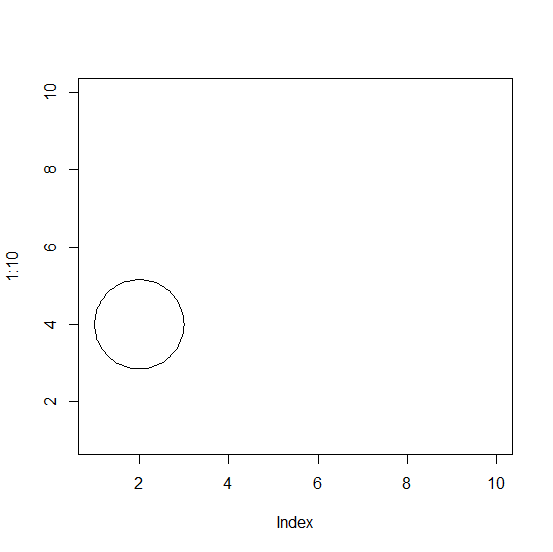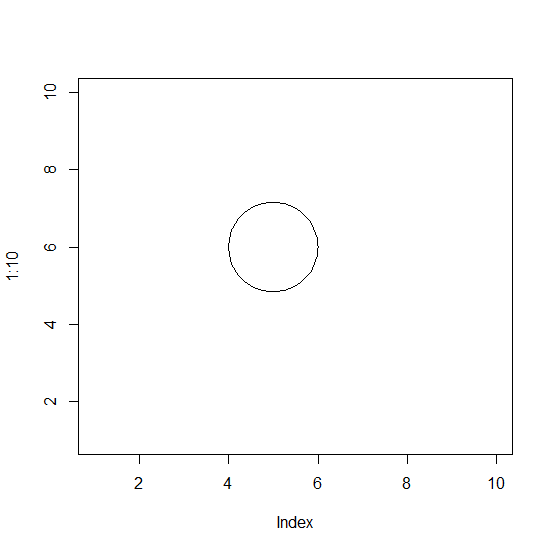# How to draw a circle in R?

There is no direct function in R to draw a circle but we can make use of plotrix package for this purpose. The plotrix package has a function called draw.cirlce which is can be used to draw a circle but we first need to draw a plot in base R then pass the correct arguments in draw.circle. The first and second arguments of draw.circle takes x and y coordinates, and the third one is for radius, hence these should be properly chosen based on the chart in base R.

> library(plotrix)

Creating different circles using draw.circle:

## Example

Live Demo

> plot(1:10,type="n")
> draw.circle(2,4,1)

## Output:## Example

Live Demo

> plot(1:10,type="n")
> draw.circle(5,6,1)

## Output: